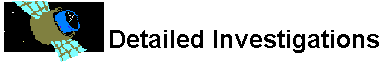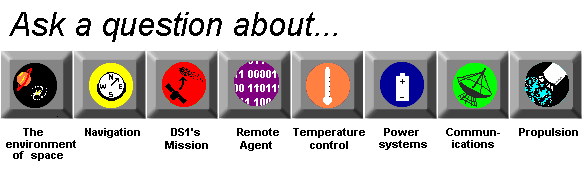# Power System

## What are radioisotope thermoelectric generators?

A radioisotope thermoelectric generator, or RTG, uses the fact that radioactive materials (such as plutonium) generate heat as they decay into non-radioactive materials. The heat used is converted into electricity by an array of thermocouples which then power the spacecraft.

A thermocouple is a device which converts thermal energy directly into electrical energy. Basically, it is made of two kinds of metal that can both conduct electricity. They are connected to each other in a closed loop. If the two metals are at different temperatures, an electric potential will exist between them. When an electric potential occurs, electrons will start to flow, making electric current.

This is a much different technique than that used by nuclear power stations on Earth. That process is called fission,and gets very high efficiency rates by literally "splitting" unstable radioactive materials (such as uranium) into smaller parts. Fission generates very large amounts of heat, but is much more complex and not as reliable as simply using the heat produced by radioactive decay. Basically, fission gives you a huge release of energy and uses fuel rapidly. An RTG gives a steadier and much smaller amount of energy.

DS1 does not use RTG's.Are RTGs safe?Where does DS1 get its electricity?How does DS1's electrical system work?What is heat?What's electron flow?What are the fuels for RTGs?What's a semiconductor?Where does energy come from and go?What's a resistor?